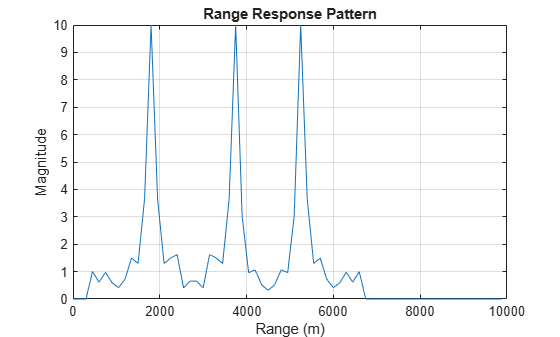# plotResponse

Plot range response from pulse compression library

## Syntax

``plotResponse(complib,X,idx)``
``plotResponse(___,pulseidx)``
``plotResponse(___,'Unit',unit)``

## Description

example

````plotResponse(complib,X,idx)` plots the range response of the input waveform, `X`, using the `idx` processing specification.```
````plotResponse(___,pulseidx)` also specifies the index, `pulseidx`, of the pulse to plot.```
````plotResponse(___,'Unit',unit)` plots the response in the units specified by `unit`.```

## Examples

collapse all

Plot the range response of an LFM signal hitting three targets at ranges of 2000, 4000, and 5500 meters. Assuming the maximum range of the radar is 10 km, determine the pulse repetition interval from the maximum range.

```% Create the pulse waveform. rmax = 10.0e3; c = physconst('Lightspeed'); pri = 2*rmax/c; fs = 1e6; pri = ceil(pri*fs)/fs; prf = 1/pri; nsamp = pri*fs; rxdata = zeros(nsamp,1); t1 = 2*2000/c; t2 = 2*4000/c; t3 = 2*5500/c; idx1 = floor(t1*fs); idx2 = floor(t2*fs); idx3 = floor(t3*fs); lfm = phased.LinearFMWaveform('PulseWidth',10/fs,'PRF',prf, ... 'SweepBandwidth',(30*fs)/40); w = lfm(); %% % Imbed the waveform part of the pulse into the received signal. x = w(1:11); rxdata(idx1:idx1+10) = x; rxdata(idx2:idx2+10) = x; rxdata(idx3:idx3+10) = x; %% % Create the pulse waveform library. w1 = {'LinearFM','PulseWidth',10/fs,'PRF',prf,... 'SweepBandwidth',(30*fs)/40}; wavlib = pulseWaveformLibrary('SampleRate',fs,'WaveformSpecification',{w1}); wav = wavlib(1); %% % Generate the range response signal. p1 = {'MatchedFilter','Coefficients',getMatchedFilter(wavlib,1),'SpectrumWindow','None'}; idx = 1; complib = pulseCompressionLibrary( ... 'WaveformSpecification',{w1}, ... 'ProcessingSpecification',{p1}, ... 'SampleRate',fs, ... 'PropagationSpeed',c); y = complib(rxdata,1); %% % Plot range response of processed data plotResponse(complib,rxdata,idx,'Unit','mag');```## Input Arguments

collapse all

Pulse compression library, specified as a `phased.PulseCompressionLibrary` System object .

Input signal, specified as a complex-valued K-by-L matrix, complex-valued K-by-N matrix, or a complex-valued K-by-N-by-L array. K denotes the number of fast time samples, L the number of pulses, and N is the number of channels. Channels can be array elements or beams.

Data Types: `double`
Complex Number Support: Yes

Index of processing specification in the pulse waveform library, specified as a positive integer.

Example: `3`

Data Types: `double`

Stepped FM waveform subpulse, specified as a positive integer. This index selects which subpulses of a stepped-FM waveform to plot. This argument only applies to stepped-FM waveforms.

Example: `5`

Data Types: `double`

Plot units, specified as `'db'`, `'mag'`, or `'pow'`. who

• `'db'` – plot the response power in dB.

• `'mag'` – plot the magnitude of the response.

• `'pow'` – plot the response power.

Example: `'mag'`

Data Types: `char` | `string`

Introduced in R2018b